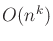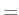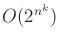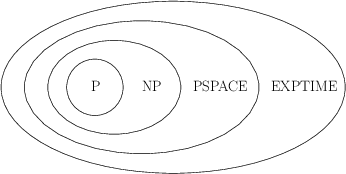#### Languages

A language is a set of binary strings associated with a problem. It represents the complete set of instances of a problem. An algorithm is said to decide a language if in finite time it correctly accepts all strings that belong to it and rejects all others. The interesting question is: How much time or space is required to decide a language? This question is asked of the problem, under the assumption that the best possible algorithm would be used to decide it. (We can easily think of inefficient algorithms that waste resources.)

A complexity class is a set of languages that can all be decided within some specified resource bound. The class P is the set of all languages (and hence problems) for which a polynomial-time algorithm exists (i.e., the algorithm runs in timefor some integer). By definition, an algorithm is called efficient if it decides its associated language in polynomial time.6.7 If no efficient algorithm exists, then the problem is called intractable. The relationship between several other classes that often emerge in theoretical motion planning is shown in Figure 6.40. The class NP is the set of languages that can be solved in polynomial time by a nondeterministic Turing machine. Some discussion of nondeterministic machines appears in Section 11.3.2. Intuitively, it means that solutions can be verified in polynomial time because the machine magically knows which choices to make while trying to make the decision. The class PSPACE is the set of languages that can be decided with no more than a polynomial amount of storage space during the execution of the algorithm (NPSPACEPSPACE, so there is no nondeterministic version). The class EXPTIME is the set of languages that can be decided in timefor some integer. It is known that EXPTIME is larger than P, but it is not known precisely where the boundaries of NP and PSPACE lie. It might be the case that P = NP = PSPACE (although hardly anyone believes this), or it could be that NP = PSPACE = EXPTIME.Steven M LaValle 2020-08-14